Get inspired by the success stories of our students in IIT JAM MS, ISI  MStat, CMI MSc DS.  Learn More

# Maximum Likelihood Estimation | ISI MStat 2017 PSB Problem 8This problem based on Maximum Likelihood Estimation, gives a detailed solution to ISI M.Stat 2017 PSB Problem 8, with a tinge of simulation and code.

## Problem

Let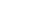be an unknown parameter, and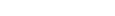be a random sample from the distribution with density.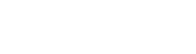Find the maximum likelihood estimator of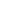and its mean squared error.

### Prerequisites

• Proof algorithm to find the MLE offor U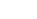• Order Statistics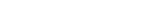• Mean Square Error

## Solution

Do you remember the method of finding the MLE offor U? Just proceed along a similar line.Let's draw the diagram.

Thus, you can see that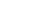is maximized at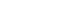.

Hence,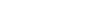.

#### MSE

Now, we need to find the distribution of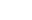.

For, that we need to find the distribution function of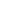.

Observe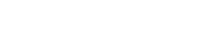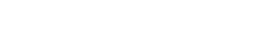MSE() = E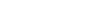=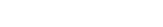=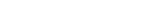=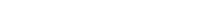=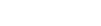+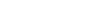-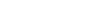=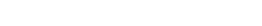Observe that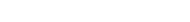.

### Let's add a computing dimension to it and verify it by simulation.

Let's take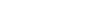. MSE is expected to be around 0.002. You can change theand n and play around.

v = NULL
n = 15
theta = 1
for (i in 1:1000) {
r = runif(n, 0, theta)
s = theta*sqrt(r) #We use Inverse Transformation Method to generate the random variables from the distribution.
m = max(s)
v = c(v,m)
}
hist(v, freq = FALSE)
k = replicate(1000,1)
mse(v,k) =  0.001959095

You should also check out this link: Triangle Inequality Problems and Solutions

I hope that helps you. Stay tuned.

This problem based on Maximum Likelihood Estimation, gives a detailed solution to ISI M.Stat 2017 PSB Problem 8, with a tinge of simulation and code.

## Problem

Letbe an unknown parameter, andbe a random sample from the distribution with density.Find the maximum likelihood estimator ofand its mean squared error.

### Prerequisites

• Proof algorithm to find the MLE offor U• Order Statistics• Mean Square Error

## Solution

Do you remember the method of finding the MLE offor U? Just proceed along a similar line.Let's draw the diagram.

Thus, you can see thatis maximized at.

Hence,.

#### MSE

Now, we need to find the distribution of.

For, that we need to find the distribution function of.

ObserveMSE() = E====+-=Observe that.

### Let's add a computing dimension to it and verify it by simulation.

Let's take. MSE is expected to be around 0.002. You can change theand n and play around.

v = NULL
n = 15
theta = 1
for (i in 1:1000) {
r = runif(n, 0, theta)
s = theta*sqrt(r) #We use Inverse Transformation Method to generate the random variables from the distribution.
m = max(s)
v = c(v,m)
}
hist(v, freq = FALSE)
k = replicate(1000,1)
mse(v,k) =  0.001959095

You should also check out this link: Triangle Inequality Problems and Solutions

I hope that helps you. Stay tuned.

This site uses Akismet to reduce spam. Learn how your comment data is processed.

### Knowledge Partner